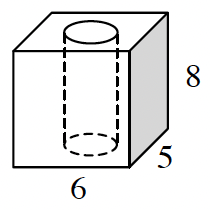### Home > GC > Chapter 10 > Lesson 10.1.1 > Problem10-6

10-6.A rectangular prism has a cylindrical hole removed, as shown at right. If the radius of the cylindrical hole is $2$ inches, find the volume and total surface area of the solid.

Find the volume of the cube.

Find the volume of the cylinder within the cube.

Subtract the volume of the cylinder from the volume of the cube.

$139.5 \; \text{in}^3$

Find the surface area of the cube.

Find the surface area of the cylinder.

Add the surface area of the cube and the lateral area of the cylinder, but subtract the area of the two holes (circles).

$311.4 \; \text{in}^2$